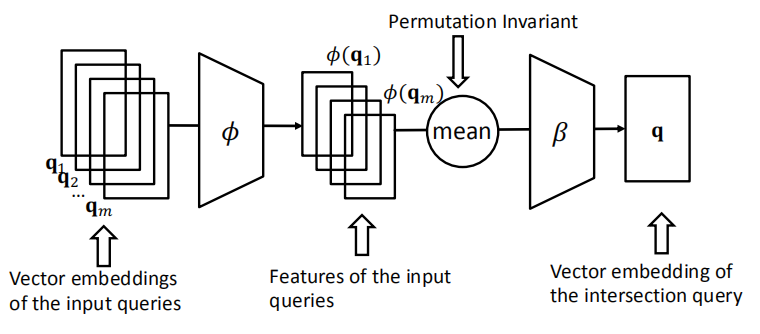# Stanford图机器学习公开课CS224W（十七）笔记

## Lecture 17 -Reasoning over Knowledge Graphs

Posted by Yunlongs on December 16, 2020

# Reasoning over Knowledge Graphs

## 一.Knowledge Graph completion

### 1.1 KG Representation

• 将KG中的实体和关系建模到同一个向量空间$\Reals ^d$
• 给定一个正确的三元组(h,r,t)，我们的目标为对于(h,r)的embedding，应当和t的embedding足够接近。

### 1.2 Relation Patterns

• Symmetric Relations:
$r(h,t)\rArr r(t,h) \ \ \ \ \ \ \ \ \forall h,t$

• Composition Relations:
$r_1(x,y) \land r_2(y,z)\rArr r_3(x,z) \ \ \ \ \ \forall x,y,z$

• 1-to-N,N-to-1 Relations：
$r(h,t_1),r(h,t_2),...,r(h,t_n)$

### 1.3 TransE

$h + r =t$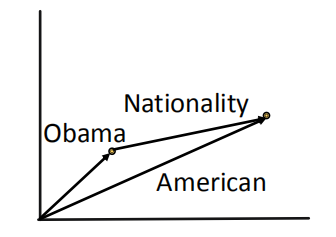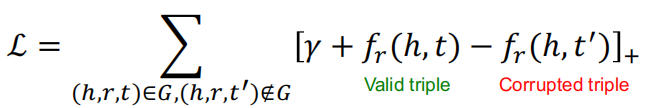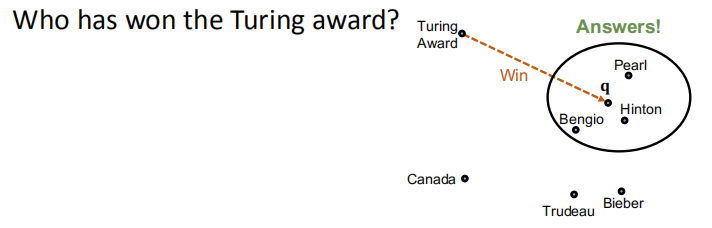TransE的局限性：Symmetric Relations 我们期望

$r(h,t)\rArr r(t,h) \ \ \ \ \ \ \ \ \forall h,t$

TransE的局限性：N-ary Relations 我们期望$(h,r,t_1)$和$(h,r,t_2)$在KG中成立，但是$t_1 = h+r = t_2$，对于相同的h和r得到的t总是相同的，但他们应当是向量空间中不同的两个实体。

### 1.4 TransR

TransR将实体建模到实体空间$\Reals ^d$的向量中，将关系建模到关系空间$\Reals ^k$的向量中去，然后使用一个投影矩阵$M_r \in \Reals ^{k \times d}$来将实体从实体空间映射到关系空间中去。

$h_{\perp}=M_{r} h, t_{\perp}=M_{r} t$

$f_r(h,t) = \|h_ \perp + r - t_ \perp \|$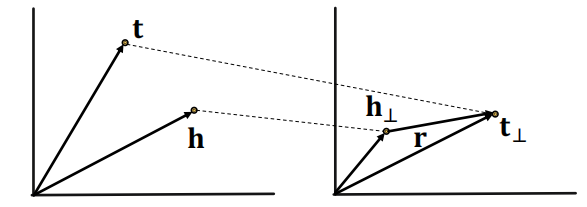$r(h,t)\rArr r(t,h) \ \ \ \ \ \ \ \ \forall h,t$

$r=0,\ h_ \perp = M_rh = M_rt = t_ \perp$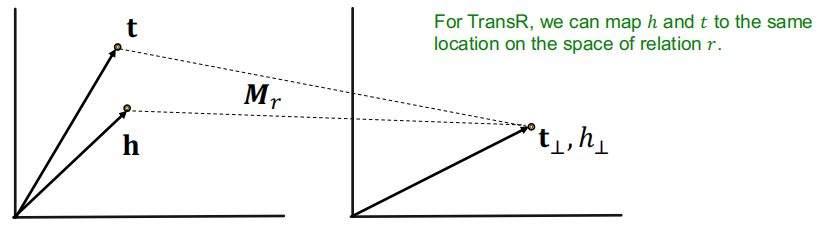TransR可以容易的处理N元关系

$t_ \perp = M_rt_1 = M_rt_2$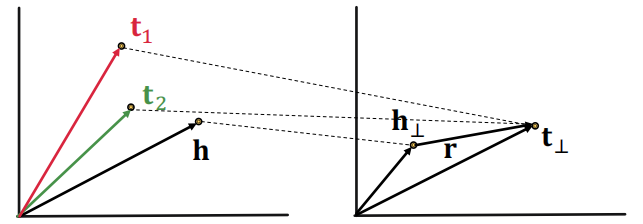$r_1(x,y) \land r_2(y,z)\rArr r_3(x,z) \ \ \ \ \ \forall x,y,z$

## 二.Path Queries

$q = (v_a,r_1,...,r_n)$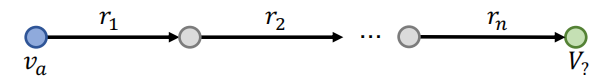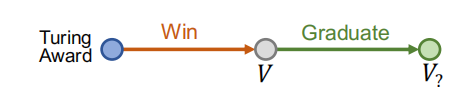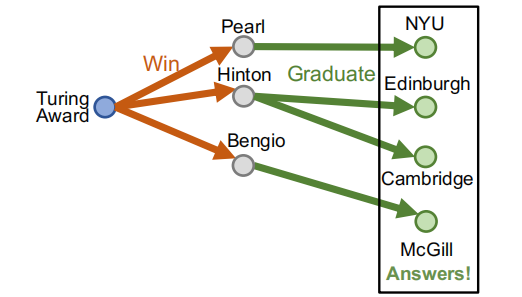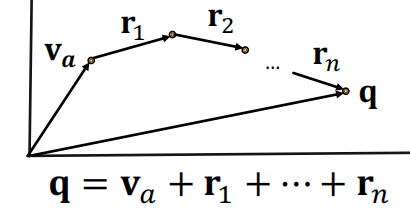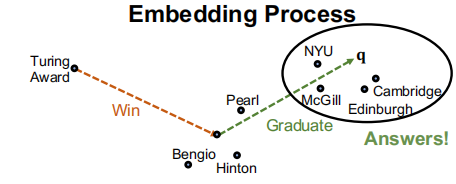## 三.Conjunctive Queries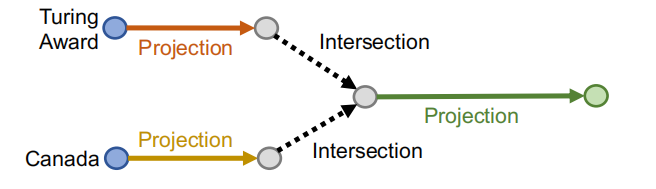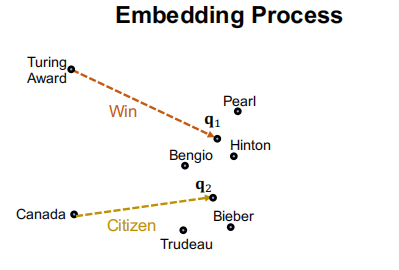• 输入当前的查询向量$q_1,…,q_m$
• 输出：查询向量q的交集
• $J$应当是置换不变的，因为查询的关系先后顺序和结果无关。即 $\mathcal{J}\left(\mathbf{q}_{1}, \ldots, \mathbf{q}_{m}\right)=\mathcal{J}\left(\mathbf{q}_{p(1)}, \ldots, \mathbf{q}_{p(m)}\right)$